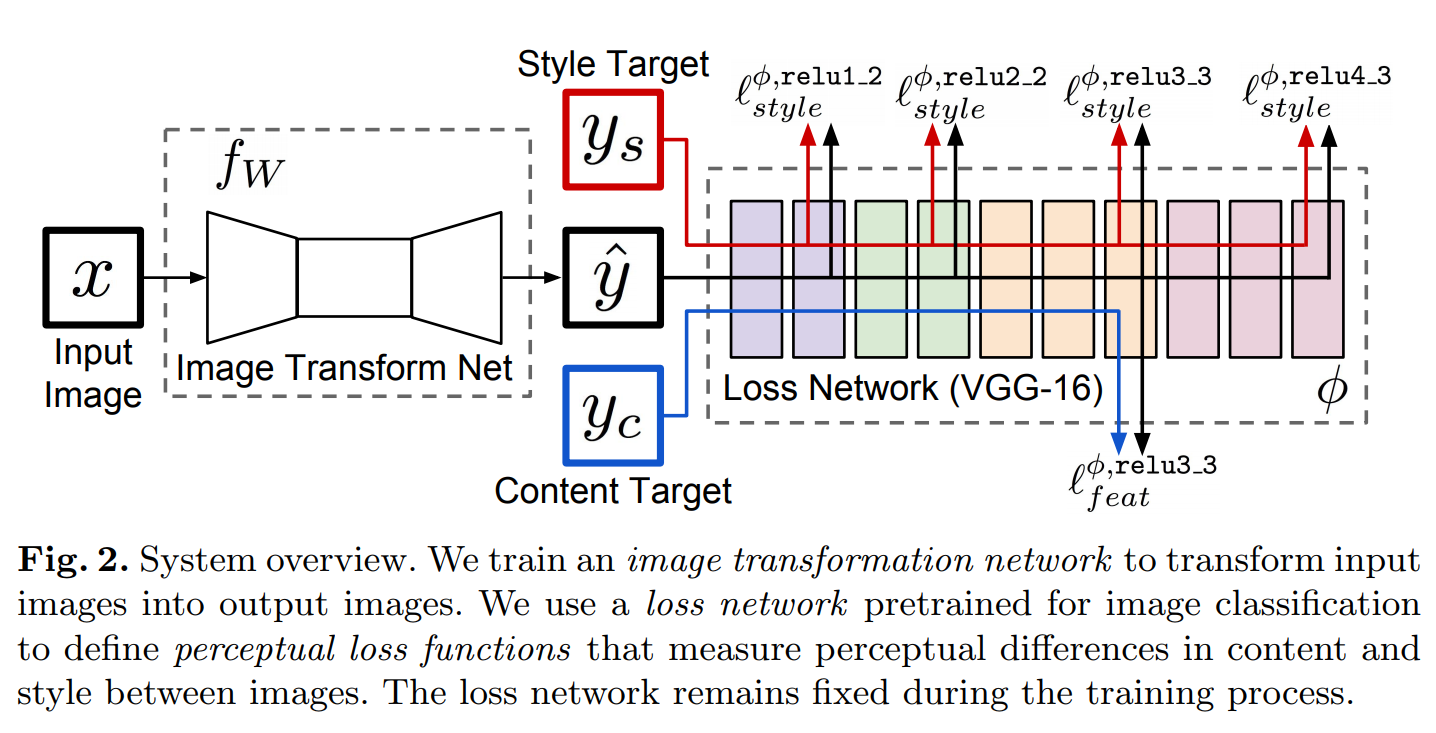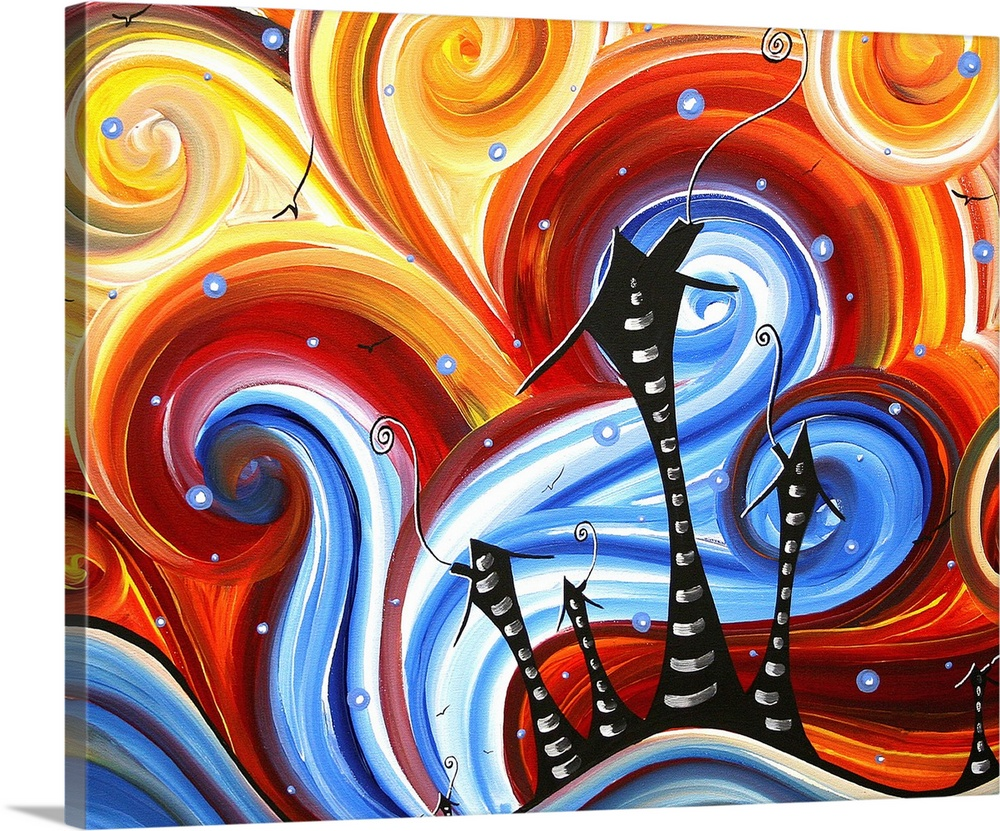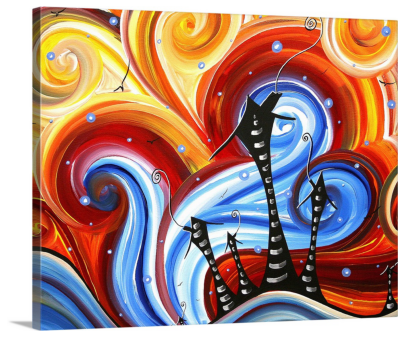/mnt/d/lib/python3.7/site-packages/torch/cuda/__init__.py:52: UserWarning: CUDA initialization: Found no NVIDIA driver on your system. Please check that you have an NVIDIA GPU and installed a driver from http://www.nvidia.com/Download/index.aspx (Triggered internally at  /pytorch/c10/cuda/CUDAFunctions.cpp:100.)


## Lesson Video:

This article is also a Jupyter Notebook available to be run from the top down. There will be code snippets that you can then run in any environment.

Below are the versions of fastai, fastcore, wwf, and nbdev currently running at the time of writing this:

• fastai: 2.2.5
• fastcore: 1.3.19
• wwf: 0.0.10
• nbdev: 1.1.12

Before we run anything we want to ensure we have a P100 GPU or better:

!nvidia-smi

Fri Feb  5 01:42:56 2021
+-----------------------------------------------------------------------------+
| NVIDIA-SMI 460.39       Driver Version: 418.67       CUDA Version: 10.1     |
|-------------------------------+----------------------+----------------------+
| GPU  Name        Persistence-M| Bus-Id        Disp.A | Volatile Uncorr. ECC |
| Fan  Temp  Perf  Pwr:Usage/Cap|         Memory-Usage | GPU-Util  Compute M. |
|                               |                      |               MIG M. |
|===============================+======================+======================|
|   0  Tesla P100-PCIE...  Off  | 00000000:00:04.0 Off |                    0 |
| N/A   38C    P0    26W / 250W |      0MiB / 16280MiB |      0%      Default |
|                               |                      |                 ERR! |
+-------------------------------+----------------------+----------------------+

+-----------------------------------------------------------------------------+
| Processes:                                                                  |
|  GPU   GI   CI        PID   Type   Process name                  GPU Memory |
|        ID   ID                                                   Usage      |
|=============================================================================|
|  No running processes found                                                 |
+-----------------------------------------------------------------------------+


## What will we cover?

• Custom loss functions
• Custom models
• Utilizing the nb_dev library
• Deployment Code

This notebook is largely based on the work by Lucas Vazquez on the fastai forum

## Intro to nbdev

• Library by Jeremy and Sylvain for writing libraries
• Used to make the entire fastai library
• Converts a ipynb to documentation as well as .py with only specific cells

• Few base terminology:

• #default_exp
• #hide
• #export

We always need the showdoc to export

## fastai libraries

from fastai.vision.all import *


## What is Style Transfer?

• Feature Loss
• Aka perception loss
• Image Transformation network
• Input images -> Output images
• Pre-Trained Loss Network
• Perceptual loss functions
• A measurement of the differences in content and style between two images## Our Pre-Trained Network:

• vgg-19:
• Small CNN pre-trained on ImageNet
• Only 19 layers deep
from torchvision.models import vgg19, vgg16

feat_net = vgg19(pretrained=True).features.cuda().eval()

Downloading: "https://download.pytorch.org/models/vgg19-dcbb9e9d.pth" to /root/.cache/torch/hub/checkpoints/vgg19-dcbb9e9d.pth


We'll get rid of the head and use the internal activations (and our generator model's loss). As a result, we want to set every layer to un-trainable

for p in feat_net.parameters(): p.requries_grad=False


We will be using feature detections that our model picks up, which is like our heatmaps generated for our Classification models

layers = [feat_net[i] for i in [1, 6, 11, 20, 29, 22]]; layers

[ReLU(inplace=True),
ReLU(inplace=True),
ReLU(inplace=True),
ReLU(inplace=True),
ReLU(inplace=True),
ReLU(inplace=True)]

The outputs are ReLU layers. Below is a configuration for the 16 and 19 models

_vgg_config = {
'vgg16' : [1, 11, 18, 25, 20],
'vgg19' : [1, 6, 11, 20, 29, 22]
}


Let's write a quick get_layers function to grab our network and the layers

def _get_layers(arch:str, pretrained=True):
"Get the layers and arch for a VGG Model (16 and 19 are supported only)"
feat_net = vgg19(pretrained=pretrained).cuda() if arch.find('9') > 1 else vgg16(pretrained=pretrained).cuda()
config = _vgg_config.get(arch)
features = feat_net.features.cuda().eval()
return feat_net, [features[i] for i in config]


Now let's make it all in one go utilizing our private functions to pass in an architecture name and a pretrained parameter

#### get_feats[source]

get_feats(arch:str, pretrained=True)

Get the features of an architecture

def get_feats(arch:str, pretrained=True):
"Get the features of an architecture"
feat_net, layers = _get_layers(arch, pretrained)
hooks = hook_outputs(layers, detach=False)
def _inner(x):
feat_net(x)
return hooks.stored
return _inner

feats = get_feats('vgg19')


## The Loss Function

Our loss fuction needs:

• Our original image
• Some artwork / style
• Activation features from our encoder

What image will we be using?Let's grab the image

url = 'https://static.greatbigcanvas.com/images/singlecanvas_thick_none/megan-aroon-duncanson/little-village-abstract-art-house-painting,1162125.jpg'

!wget {url} -O 'style.jpg'

--2021-02-05 01:43:21--  https://static.greatbigcanvas.com/images/singlecanvas_thick_none/megan-aroon-duncanson/little-village-abstract-art-house-painting,1162125.jpg
Resolving static.greatbigcanvas.com (static.greatbigcanvas.com)... 3.86.0.166, 52.205.76.115
Connecting to static.greatbigcanvas.com (static.greatbigcanvas.com)|3.86.0.166|:443... connected.
HTTP request sent, awaiting response... 200 OK
Length: 484954 (474K) [image/jpeg]
Saving to: ‘style.jpg’

style.jpg           100%[===================>] 473.59K  1.80MB/s    in 0.3s

2021-02-05 01:43:22 (1.80 MB/s) - ‘style.jpg’ saved [484954/484954]


fn = 'style.jpg'


We can now make a PipeLine to convert our image into a Tensor to use in our loss function. We'll want to use the Datasets for this

dset = Datasets(fn, tfms=[PILImage.create])

dl = dset.dataloaders(after_item=[ToTensor()], after_batch=[IntToFloatTensor(), Normalize.from_stats(*imagenet_stats)], bs=1)

dl.show_batch(figsize=(7,7))style_im = dl.one_batch()

style_im.shape

(1, 3, 831, 1000)

#### get_style_im[source]

get_style_im(url)

def get_style_im(url):
fn = 'style.jpg'
dset = Datasets(fn, tfms=[PILImage.create])
dl = dset.dataloaders(after_item=[ToTensor()], after_batch=[IntToFloatTensor(), Normalize.from_stats(*imagenet_stats)], bs=1)
return dl.one_batch()


We can then grab the features using our feats function we made earlier

im_feats = feats(style_im)


Let's look at their sizes

for feat in im_feats:
print(feat.shape)

(1, 64, 831, 1000)
(1, 128, 415, 500)
(1, 256, 207, 250)
(1, 512, 103, 125)
(1, 512, 51, 62)
(1, 512, 103, 125)


Now we can bring those images down to the channel size

#### gram[source]

gram(x:Tensor)

Transpose a tensor based on c,w,h

def gram(x:Tensor):
"Transpose a tensor based on c,w,h"
n, c, h, w = x.shape
x = x.view(n, c, -1)
return (x @ x.transpose(1, 2))/(c*w*h)

im_grams = [gram(f) for f in im_feats]

for feat in im_grams:
print(feat.shape)

(1, 64, 64)
(1, 128, 128)
(1, 256, 256)
(1, 512, 512)
(1, 512, 512)
(1, 512, 512)


#### get_stl_fs[source]

get_stl_fs(fs)

def get_stl_fs(fs): return fs[:-1]


We're almost there! Let's look at why that was important

#### style_loss[source]

style_loss(inp:Tensor, out_feat:Tensor)

Calculate style loss, assumes we have im_grams

def style_loss(inp:Tensor, out_feat:Tensor):
"Calculate style loss, assumes we have im_grams"
# Get batch size
bs = inp.shape
loss = []
# For every item in our inputs
for y, f in zip(*map(get_stl_fs, [im_grams, inp])):
# Calculate MSE
loss.append(F.mse_loss(y.repeat(bs, 1, 1), gram(f)))
# Multiply their sum by 30000
return 3e5 * sum(loss)


Great, so what now? Let's make a loss function for fastai!

• Remember, we do not care to use any initial metrics

## classFeatureLoss[source]

FeatureLoss(feats, style_loss, act_loss) :: Module

Combines two losses and features into a useable loss function

class FeatureLoss(Module):
"Combines two losses and features into a useable loss function"
def __init__(self, feats, style_loss, act_loss):
store_attr()
self.reset_metrics()

def forward(self, pred, targ):
# First get the features of our prediction and target
pred_feat, targ_feat = self.feats(pred), self.feats(targ)
# Calculate style and activation loss
style_loss = self.style_loss(pred_feat, targ_feat)
act_loss = self.act_loss(pred_feat, targ_feat)
# Store the loss
# Return the sum
return style_loss + act_loss

def reset_metrics(self):
# Generates a blank metric
self.metrics = dict(style = [], content = [])

self.metrics['style'].append(style_loss)
self.metrics['content'].append(act_loss)


#### act_loss[source]

act_loss(inp:Tensor, targ:Tensor)

Calculate the MSE loss of the activation layers

def act_loss(inp:Tensor, targ:Tensor):
"Calculate the MSE loss of the activation layers"
return F.mse_loss(inp[-1], targ[-1])


Let's declare our loss function by passing in our features and our two 'mini' loss functions

loss_func = FeatureLoss(feats, style_loss, act_loss)


## The Model Architecture

Let's now build our model

## classReflectionLayer[source]

ReflectionLayer(in_channels, out_channels, ks=3, stride=2) :: Module

A series of Reflection Padding followed by a ConvLayer

class ReflectionLayer(Module):
"A series of Reflection Padding followed by a ConvLayer"
def __init__(self, in_channels, out_channels, ks=3, stride=2):
self.conv2d = nn.Conv2d(in_channels, out_channels, ks, stride)

def forward(self, x):
out = self.conv2d(out)
return out

ReflectionLayer(3, 3)

ReflectionLayer(
(conv2d): Conv2d(3, 3, kernel_size=(3, 3), stride=(2, 2))
)

## classResidualBlock[source]

ResidualBlock(channels) :: Module

Two reflection layers and an added activation function with residual

class ResidualBlock(Module):
"Two reflection layers and an added activation function with residual"
def __init__(self, channels):
self.conv1 = ReflectionLayer(channels, channels, ks=3, stride=1)
self.in1 = nn.InstanceNorm2d(channels, affine=True)
self.conv2 = ReflectionLayer(channels, channels, ks=3, stride=1)
self.in2 = nn.InstanceNorm2d(channels, affine=True)
self.relu = nn.ReLU()

def forward(self, x):
residual = x
out = self.relu(self.in1(self.conv1(x)))
out = self.in2(self.conv2(out))
out = out + residual
return out

ResidualBlock(3)

ResidualBlock(
(conv1): ReflectionLayer(
(conv2d): Conv2d(3, 3, kernel_size=(3, 3), stride=(1, 1))
)
(in1): InstanceNorm2d(3, eps=1e-05, momentum=0.1, affine=True, track_running_stats=False)
(conv2): ReflectionLayer(
(conv2d): Conv2d(3, 3, kernel_size=(3, 3), stride=(1, 1))
)
(in2): InstanceNorm2d(3, eps=1e-05, momentum=0.1, affine=True, track_running_stats=False)
(relu): ReLU()
)

## classUpsampleConvLayer[source]

UpsampleConvLayer(in_channels, out_channels, ks=3, stride=1, upsample=None) :: Module

Upsample with a ReflectionLayer

class UpsampleConvLayer(Module):
"Upsample with a ReflectionLayer"
def __init__(self, in_channels, out_channels, ks=3, stride=1, upsample=None):
self.upsample = upsample
self.conv2d = nn.Conv2d(in_channels, out_channels, ks, stride)

def forward(self, x):
x_in = x
if self.upsample:
x_in = torch.nn.functional.interpolate(x_in, mode='nearest', scale_factor=self.upsample)
out = self.conv2d(out)
return out


Let's put everything together into a model

## classTransformerNet[source]

TransformerNet() :: Module

A simple network for style transfer

class TransformerNet(Module):
"A simple network for style transfer"
def __init__(self):
# Initial convolution layers
self.conv1 = ReflectionLayer(3, 32, ks=9, stride=1)
self.in1 = nn.InstanceNorm2d(32, affine=True)
self.conv2 = ReflectionLayer(32, 64, ks=3, stride=2)
self.in2 = nn.InstanceNorm2d(64, affine=True)
self.conv3 = ReflectionLayer(64, 128, ks=3, stride=2)
self.in3 = nn.InstanceNorm2d(128, affine=True)
# Residual layers
self.res1 = ResidualBlock(128)
self.res2 = ResidualBlock(128)
self.res3 = ResidualBlock(128)
self.res4 = ResidualBlock(128)
self.res5 = ResidualBlock(128)
# Upsampling Layers
self.deconv1 = UpsampleConvLayer(128, 64, ks=3, stride=1, upsample=2)
self.in4 = nn.InstanceNorm2d(64, affine=True)
self.deconv2 = UpsampleConvLayer(64, 32, ks=3, stride=1, upsample=2)
self.in5 = nn.InstanceNorm2d(32, affine=True)
self.deconv3 = ReflectionLayer(32, 3, ks=9, stride=1)
# Non-linearities
self.relu = nn.ReLU()

def forward(self, X):
y = self.relu(self.in1(self.conv1(X)))
y = self.relu(self.in2(self.conv2(y)))
y = self.relu(self.in3(self.conv3(y)))
y = self.res1(y)
y = self.res2(y)
y = self.res3(y)
y = self.res4(y)
y = self.res5(y)
y = self.relu(self.in4(self.deconv1(y)))
y = self.relu(self.in5(self.deconv2(y)))
y = self.deconv3(y)
return y

net = TransformerNet()


We will be using the COCO_SAMPLE dataset

path = untar_data(URLs.COCO_SAMPLE)


Our DataBlock needs to be Image -> Image

dblock = DataBlock(blocks=(ImageBlock, ImageBlock),
get_items=get_image_files,
splitter=RandomSplitter(0.1, seed=42),
item_tfms=[Resize(224)],
batch_tfms=[Normalize.from_stats(*imagenet_stats)])


If you do not pass in a get_y, fastai will assume your input = output

dls = dblock.dataloaders(path, bs=22)

dls.show_batch()